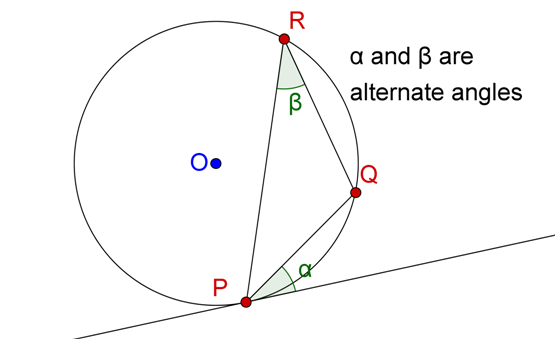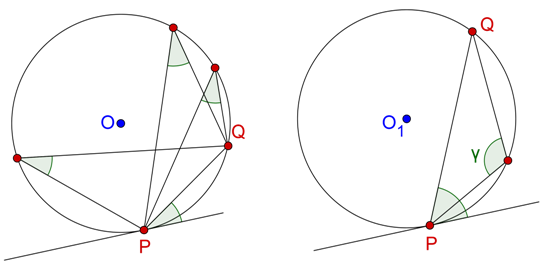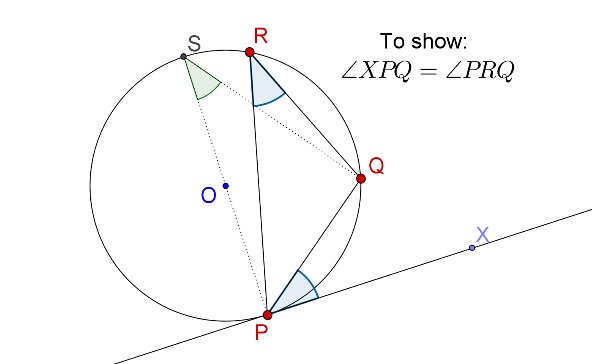# Alternate Segment Theorem

Go back to  'Circles-Tangents'

Suppose that you drawn a tangent to a circle such that the point of contact is P, and through P, you draw a chord PQ, which is inclined to the tangent at an angle $$\alpha$$. Also, suppose that PQ subtends an angle $$\beta$$ at a point R anywhere on the circumference of the circle, as shown:We will call the angle PRQ, that is, $$\angle \beta$$ , the alternate angle for the angle between the tangent at P and the chord PQ. In other words, $$\angle \alpha$$ and $$\angle \beta$$ are alternate angles with respect to the tangent at P and the chord PQ. Note that this angle will be the same regardless of the position of R (as long as R stays in the segment opposite the tangent). To understand this better, consider the figure below. On the left, you have three valid alternate angles (and all of them will be equal) for the tangent at P and the chord PQ, whereas on the right, the angle marked $$\gamma$$ is not the alternate angle for the tangent at P, since it is on the same side of the chord PQ as the tangent at P.The following theorem is a very important result on alternate angles.

Theorem: For any circle, the angle between a tangent and a chord through the point of contact of the tangent is equal to the alternate segment.

Try to prove this on your own before proceeding further.

Proof: The proof is straightforward. Consider the following figure:PS is the diameter through P. We note that $$\angle {\rm{PQS }} = {\rm{ }}{90^0}$$ . Also, $$\angle {\rm{PSQ }} = \angle {\rm{PRQ}}$$ , since they are angles in the same segment. Now,

\begin{align} &{\angle PSQ{\rm{ }} + \angle QPS{\rm{ }} = {\rm{ }}{{90}^0}}\\&{\angle XPQ{\rm{ }} + \angle QPS{\rm{ }} = {\rm{ }}{{90}^0}} \end{align}

Thus,

$$\angle {\rm{PSQ }} + \angle {\rm{QPS }} = \angle {\rm{XPQ }} + \angle {\rm{QPS}}$$

è$$\angle {\rm{PSQ }} = \angle {\rm{XPQ}}$$

Further, this means that $$\angle {\rm{XPQ }} = \angle {\rm{PRQ}}$$ , since we have already seen that $$\angle {\rm{PSQ }} = \angle {\rm{PRQ}}$$. This justifies the assertion that the angle between the tangent and the chord through the point of contact is equal to the angle in the alternate segment (that is, the alternate angle).

As an exercise, construct a circle, a tangent L to the circle at a point P on the circle, and a chord PQ through P. Confirm (by actually measuring angles) that the angle between L and PQ is the same as the angle which PQ subtends at a point anywhere in the alternate segment.Circles and Tangents
grade 10 | Questions Set 1
Circles and Tangents
Circles and Tangents
grade 10 | Questions Set 2
Circles and Tangents